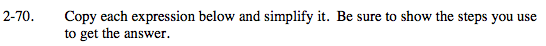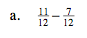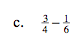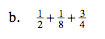### Home > MC2 > Chapter 2 > Lesson 2.2.3 > Problem2-70

2-70.Refer to problem 1-79 for help.Find a common denominator for both fractions, then use a Giant One to convert each.

Refer to problem 1-79.Find a common denominator among all three fractions.

8 is a good common denominator to use.

$\frac{4}{8} + \frac{1}{8} + \frac{6}{8}$

Refer to problem 1-79.